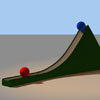#### You may also like### Lunar Leaper

Gravity on the Moon is about 1/6th that on the Earth. A pole-vaulter 2 metres tall can clear a 5 metres pole on the Earth. How high a pole could he clear on the Moon?### Which Twin Is Older?

A simplified account of special relativity and the twins paradox.### Whoosh

A ball whooshes down a slide and hits another ball which flies off the slide horizontally as a projectile. How far does it go?

# Earth Orbit

##### Age 16 to 18Challenge Level

We have to solve $$\frac{d^2r}{dt^2} - \frac{h^2}{r^3} = -\frac{GM}{r^2}\;.$$ $h = r^2\frac{d\theta}{dt}$ is constant. Putting $u = \frac{1}{r}$ we have $\frac{d\theta}{dt} = hu^2$ and $$\frac{dr}{dt} = \frac{dr}{du}\frac{du}{d\theta}\frac{d\theta}{dt} = -\frac{1}{u^2}\frac{du}{d\theta}hu^2 = -h\frac{du}{d\theta}$$ Therefore, $$\frac{d^2r}{dt^2} = -h\frac{d}{dt}\left(\frac{du}{d\theta}\right) = -h\frac{d\theta}{dt}\frac{d^2u}{d\theta^2} = -h^2u^2\frac{d^2u}{d\theta^2}\;.$$ Our differential equation now becomes $$-h^2u^2\frac{d^2u}{d\theta^2} - h^2u^3 = -GMu^2\quad \Rightarrow\quad \frac{d^2u}{d\theta^2} + u = \frac{GM}{h^2}$$ which has a general solution $u(\theta) = \frac{GM}{h^2} + A\cos{\theta} + B\sin{\theta}$ for constants $A$ and $B$.

Now we substitute back to $r$, and get $$r = \frac{1}{\frac{GM}{h^2} + A\cos{\theta} + B\sin{\theta}} = \frac{\frac{h^2}{GM}}{1 + \frac{Ah^2}{GM}\sin{\theta} + \frac{Bh^2}{GM}\cos{\theta}} = \frac{\frac{h^2}{GM}}{1 + e\cos{(\theta + f)}}$$ for some $e$ and $f$. Therefore $h^2 = GMr(1 + e\cos{(\theta + f)})$.